Gallery: Histograms

1) Displaying (x,y) data points as a histogram

Histograms are used to plot binned one-dimensional data - of the form (xmid, y) or (xlow, xhigh, y) - with optional error bars on the Y values. A later example shows how to display error bars on the points.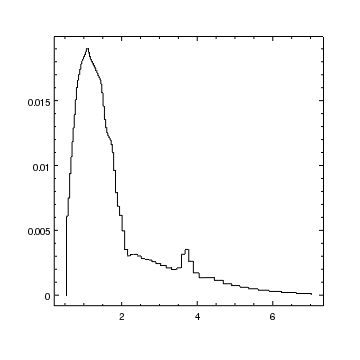Version: Postscript; PDF
add_histogram("spectrum.fits[fit][cols x,y]")


In this example the data is stored as a set of (x, y) points, where the x values give the center of each bin. Unlike curves, histograms default to only being drawn by a solid line; the symbol.style attribute is set to none.

The preferences for curves can be found by using the get_preference call:

chips> get_preference("histogram")

histogram.stem         : hist
histogram.depth        : default
histogram.line.color   : default
histogram.line.thickness: 1
histogram.line.style   : solid
histogram.symbol.color : default
histogram.symbol.style : none
histogram.symbol.size  : 5
histogram.symbol.angle : 0
histogram.symbol.fill  : false
histogram.err.color    : default
histogram.err.thickness: 1
histogram.err.style    : line
histogram.err.up       : on
histogram.err.down     : on
histogram.err.caplength: 10
histogram.dropline     : off
histogram.fill.color   : default
histogram.fill.opacity : 1
histogram.fill.style   : nofill


The settings for the current histogram can be found by using the get_histogram routine:

chips> get_histogram()

depth = 100
dropline = False
err.caplength = 10
err.color = default
err.down = False
err.style = line
err.thickness = 1.0
err.up = False
fill.color = default
fill.opacity = 1.0
fill.style = 0
id = None
line.color = default
line.style = 1
line.thickness = 1.0
stem = None
symbol.angle = 0.0
symbol.color = default
symbol.fill = False
symbol.size = 5
symbol.style = 0


2) Displaying (xlow,xhigh,y) data points as a histogram

In the first example, the binned data was given using the mid-point of each bin. In this example we show how histograms can be plotted by giving the low and high edges of each bin.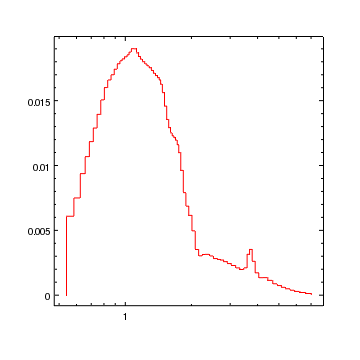Version: Postscript; PDF
tbl = read_file("spectrum.fits[fit]")
xlo = copy_colvals(tbl,"xlo")
xhi = copy_colvals(tbl,"xhi")
y = copy_colvals(tbl,"y")
log_scale(X_AXIS)


The bin edges can not be specified by passing in a file name to add_histogram, so we have to read in the arrays using crates routines and then plot them. We use the read_file to read in the file, copy_colvals to get the column values, and then add_histogram to plot them.

3) A histogram which shows the bin edges

When using xmid values, the bins are assumed to be contiguous. This is not the case when the bin edges - namely xlow and xhigh - are given. Here we plot data from a histogram with non-contiguous bins, setting the dropline attribute so that all the bin edges are drawn (this attribute can also be used with histograms like that used in the first example).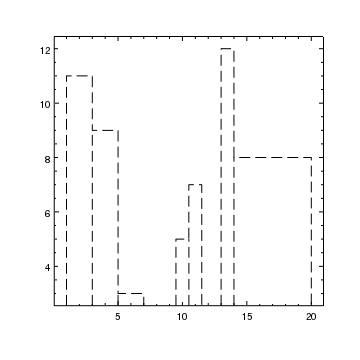Version: Postscript; PDF
tbl = read_file("histogram.fits")
xlo = copy_colvals(tbl,"xlo")
xhi = copy_colvals(tbl,"xhi")
y = copy_colvals(tbl,"y")


4) A histogram showing the full range of options

In this example we change most of the attributes of a histogram. The Filling a histogram with a pattern example shows how you can change the fill style of a histogram from solid to a pattern.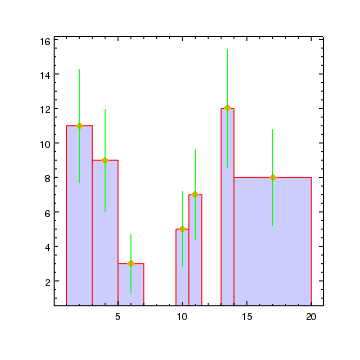Version: Postscript; PDF
tbl = read_file("histogram.fits")
xlo = copy_colvals(tbl,"xlo")
xhi = copy_colvals(tbl,"xhi")
y = copy_colvals(tbl,"y")
dylo = copy_colvals(tbl,"dylo")
dyhi = copy_colvals(tbl,"dyhi")
hist = ChipsHistogram()
hist.dropline = True
hist.line.color = "red"
hist.symbol.style = "diamond"
hist.symbol.size = 4
hist.symbol.fill = True
hist.symbol.color = "orange"
hist.err.color = "green"
hist.fill.style = "solid"
hist.fill.opacity = 0.2
hist.fill.color = "blue"

# Move the histogram behind the axes so that the tick marks are not hidden
shuffle_back(chips_histogram)


Opacity and Postscript output

Note that the postscript output created by print_window does not support opaque region or histogram fills; instead the opacity is taken to be 1. The relative depth of the objects can be changed - by altering the depth attribute or using the various "shuffle commands" (shuffle, shuffle_back, shuffle_front, shuffle_backward, shuffle_forward, and the set of shuffle_<object> routines) so that overlapping objects are not completely obscured if desired.

Solid fill and Postscript output

When using a solid fill, the off-screen output may show a pattern of lines within histograms or regions for postscript outputs, depending on what display program you are using. They should not appear when printed out.

5) Filling a histogram with a pattern

In this example we fill the histogram using a pattern, rather than a solid fill as used in the A histogram showing the full range of options example.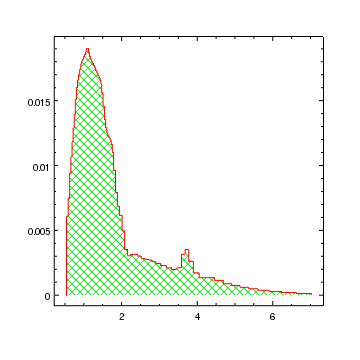Version: Postscript; PDF
add_histogram("spectrum.fits[fit][cols x,y]")
set_histogram(["fill.style","crisscross"])
set_histogram(["fill.color","green","line.color","red"])


The fill.style attribute of histograms is used to determine how the region is filled. Here we use the value "crisscross", rather than "solid", to fill the histogram with crossed lines. These lines can be colored independently of the histogram boundary.

6) Comparing two histograms

Multiple histograms can be added to a plot. Here we use a combination of the opacity setting and careful bin placement to allow the data to be compared.

The idea of this figure is to compare the Normal and Poisson distribution, calculated using the routines from the np.random module.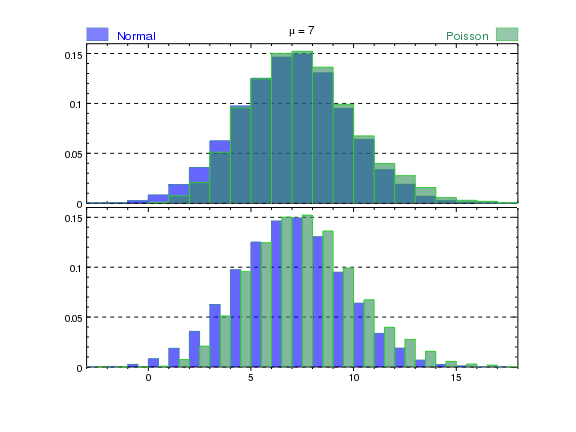Version: Postscript; PDF

def compare(mu, npts=10000):
"""Compare the Poisson and Normal distributions for an
expected value of mu, using npts points. Draws histograms
displaying the probability density function."""

ns = np.random.normal(mu, np.sqrt(mu), npts)
ps = np.random.poisson(mu, npts)

# Calculate the range of the histogram (using
# a bin width of 1). Since np.histogram needs
# the upper edge of the last bin we need edges
# to start at xmin and end at xmax+1.
xmin = np.floor(min(ns.min(), ps.min()))
xmax = np.ceil(min(ns.max(), ps.max()))
edges = np.arange(xmin, xmax+2)
xlo = edges[:-1]
xhi = edges[1:]

# Calculate the histograms (np.histogram returns
# the y values and then the edges)
h1 = np.histogram(ns, bins=edges, normed=True)
h2 = np.histogram(ps, bins=edges, normed=True)

# Set up preferences for the histograms
hprop = ChipsHistogram()
hprop.dropline = True
hprop.fill.style = "solid"
hprop.fill.opacity = 0.6

split(2, 1, 0.01)

# In the top plot we overlay the two histograms,
# relying on the opacity to show the overlaps
hprop.fill.color = "blue"
hprop.line.color = "steelblue"

hprop.fill.color = "seagreen"
hprop.line.color = "lime"

# Start the Y axis at 0, autoscale the maximum value
limits(Y_AXIS, 0, AUTO)

# Annotate the plot
set_plot_title(r"\mu = {}".format(mu))
hide_axis('ax1')
set_yaxis(['majorgrid.visible', True])

# Add regions to the title indicating the histogram type
xr = np.asarray([0, 0.05, 0.05, 0])
yr = [1.02, 1.02, 1.1, 1.1]
ropts = {'coordsys': PLOT_NORM, 'fill.color': 'blue', 'edge.color': 'steelblue'}
lopts = {'coordsys': PLOT_NORM, 'size': 16, 'valign': 0.5, 'color': 'blue'}

ropts['fill.color'] = 'seagreen'
ropts['edge.color'] = 'lime'
lopts['color'] = 'seagreen'
lopts['halign'] = 1

current_plot('plot2')
# In the bottom plot we separate the two histograms,
# so that they each cover half the bin width
hprop.fill.color = "blue"
hprop.line.color = "steelblue"

hprop.fill.color = "seagreen"
hprop.line.color = "lime"

set_yaxis(['majorgrid.visible', True])
set_xaxis(['majortick.style', 'outside', 'minortick.style', 'outside'])

limits(Y_AXIS, 0, AUTO)
bind_axes('plot1', 'ax1', 'plot2', 'ax1')
limits(X_AXIS, xmin, xmax)

compare(7)


The code is written as a routine, which takes a single argument, mu, the expected value for the two distributions. An optional argument (npts) allows the number of points used to create each distribution to be changed, but is not actually used when we call the routine with mu=7 at the end of the script.

The calls to np.random.normal and np.random.poisson create 10000 random numbers each, drawn from the given distribution (we set the sigma of the normal distribution to be the square root of the expected value). These arrays are used to determine the minimum and maximum ranges for the histograms (converted to the nearest integer) using the np.min, np.max, np.floor and np.ceil routines from numpy. From these values we can calculate the edges array used to create the histogram; see the np.histogram documentation for an explanation of the bins and normed arguments.

The visualization consists of two plots; the first with the two histograms overlain and the second has the bins split evenly within each bin.

Annotation is added to label the visualization; in particular regions and labels are added to the left and right of the title area (by using the plot-normalized coordinate system and taking advantage of the halign attribute to right-align the "Poisson" label).

Most of the examples set attributes using the "list" approach but here we either use a dictionary, where the key is the attribute name, or use the ChipsXXX object. The following would all produce a curve with no symbols and a green line:

lopts1 = ['line.color', 'green', 'symbol.style', 'none']
lopts2 = {'line.color': 'green', 'symbol.style': 'none'}
lopts3 = ChipsCurve()
lopts3.line.color = 'green'
lopts3.lsymbol.syle = 'none'